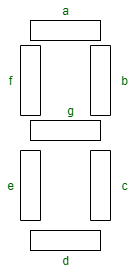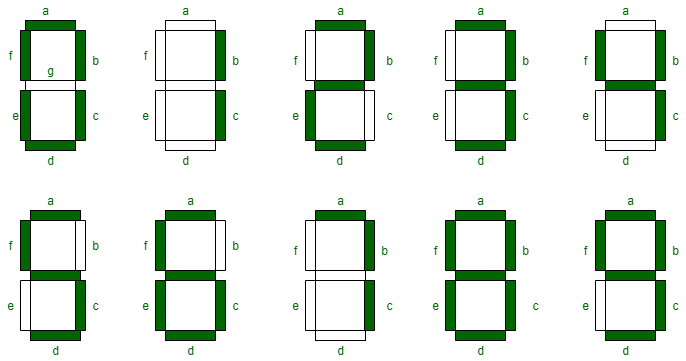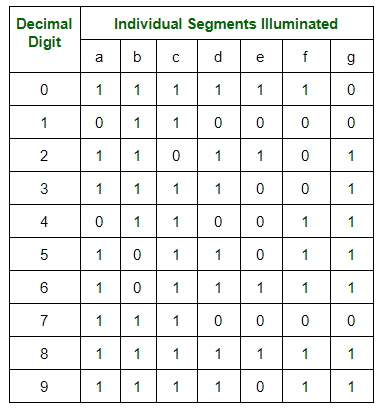# Seven Segment Displays

Light Emitting Diode (LED) is the most widely used semiconductor which emits either visible light or invisible infrared light when forward biased. Remote controls generate invisible light. A Light emitting diode (LED) is an optical electrical energy into light energy when voltage is applied.

Seven Segment Displays:
Seven segment displays are the output display device that provide a way to display information in the form of image or text or decimal numbers which is an alternative to the more complex dot matrix displays. It is widely used in digital clocks, basic calculators, electronic meters, and other electronic devices that display numerical information. It consists of seven segments of light emitting diodes (LEDs) which is assembled like numerical 8.Working of Seven Segment Displays:
The number 8 is displayed when the power is given to all the segments and if you disconnect the power for ‘g’, then it displays number 0. In a seven segment display, power (or voltage) at different pins can be applied at the same time, so we can form combinations of display numerical from 0 to 9. Since seven segment displays can not form alphabet like X and Z, so it can not be used for alphabet and it can be used only for displaying decimal numerical magnitudes. However, seven segment displays can form alphabets A, B, C, D, E, and F, so they can also used for representing hexadecimal digits.We can produce a truth table for each decimal digitTherefore, Boolean expressions for each decimal digit which requires respective light emitting diodes (LEDs) are ON or OFF. The number of segments used by digit: 0, 1, 2, 3, 4, 5, 6, 7, 8, and 9 are 6, 2, 5, 5, 4, 5, 6, 3, 7, and 6 respectively. Seven segment displays must be controlled by other external devices where different types of microcontrollers are useful to communicate with these external devices, like switches, keypads, and memory.

Types of Seven Segment Displays:
According to the type of application, there are two types of configurations of seven segment displays: common anode display and common cathode display.

1. In common cathode seven segment displays, all the cathode connections of LED segments are connected together to logic 0 or ground. We use logic 1 through a current limiting resistor to forward bias the individual anode terminals a to g.
2. Whereas all the anode connections of the LED segments are connected together to logic 1 in common anode seven segment display. We use logic 0 through a current limiting resistor to the cathode of a particular segment a to g.

Common anode seven segment displays are more popular than cathode seven segment displays, because logic circuits can sink more current than they can source and it is the same as connecting LEDs in reverse.

Applications of Seven Segment Displays:
Common applications of seven segment displays are in:

1. Digital clocks
3. Calculators
4. Wristwatchers
5. Speedometers
6. Motor-vehicle odometers